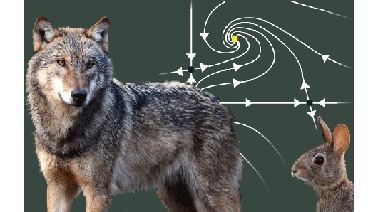# Differential Equations: 2x2 Systems

In order to understand most phenomena in the world, we need to understand not just single equations, but systems of differential equations. In this course, we start with 2x2 systems.## There is one session available:

After a course session ends, it will be archived.
Estimated 8 weeks
2–5 hours per week
Instructor-paced
Instructor-led on a course schedule

Differential equations are the language of the models we use to describe the world around us. Most phenomena require not a single differential equation, but a system of coupled differential equations. In this course, we will develop the mathematical toolset needed to understand 2x2 systems of first order linear and nonlinear differential equations. We will use 2x2 systems and matrices to model:

• predator-prey populations in an ecosystem,
• competition for tourism between two states,
• the temperature profile of a soft boiling egg,
• automobile suspensions for a smooth ride,
• pendulums, and
• RLC circuits that tune to specific frequencies.

The five modules in this seriesare being offered as an XSeries on edX. Please visit the Differential EquationsXSeries Program Page to learn more and to enroll in the modules.

• Wolf photo by Arne von Brill on Flickr (CC BY 2.0)
• Rabbit photo by Marit & Toomas Hinnosaar on Flickr (CC BY 2.0)

### At a glance

• Institution: MITx
• Subject: Math
• Level: Intermediate
• Prerequisites:

18.031x Introduction to Differential Equations (Scalar equations)

# What you'll learn

Skip What you'll learn
• How to model real world problems by 2x2 systems of differential equations
• How to use matrix methods to solve homogeneous systems of 2 first order linear differential equations
• How to use graphical methods to understand the qualitative behavior of linear and nonlinear systems, and how to apply linear approximation to nonlinear (autonomous) 2x2 systems

# Syllabus

Skip Syllabus

Unit 1: Linear 2x2 systems
1. Introduction to systems of differential equations
2. Solving 2x2 homogeneous linear systems of differential equations
3. Complex eigenvalues, phase portraits, and energy
4. The trace-determinant plane and stability

Unit 2: Nonlinear 2x2 systems

5. Linear approximation of autonomous systems
6. Stability of autonomous systems
7. Nonlinear pendulum